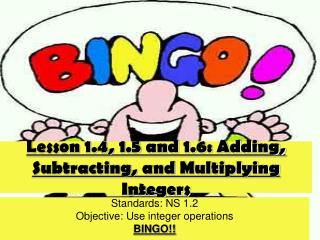DownloadDownload PresentationLesson 1.4, 1.5 and 1.6: Adding, Subtracting, and Multiplying Integers

# Lesson 1.4, 1.5 and 1.6: Adding, Subtracting, and Multiplying Integers

Download Presentation## Lesson 1.4, 1.5 and 1.6: Adding, Subtracting, and Multiplying Integers

- - - - - - - - - - - - - - - - - - - - - - - - - - - E N D - - - - - - - - - - - - - - - - - - - - - - - - - - -
##### Presentation Transcript

1. Lesson 1.4, 1.5 and 1.6: Adding, Subtracting, and Multiplying Integers Standards: NS 1.2 Objective: Use integer operations BINGO!!

2. Bingo! • The winner who says “BINGO” at the appropriate time will get a homework pass! • You will be playing black out, so every square must be marked to win!!! • Anyone acting poorly will be disqualified.

3. Numbers • Pick some of the numbers to place in any empty box. These numbers represent possible answers to problems. -41, -39, -36, -16, -14, -12, -11, -9, -7, -6 -5, -3, -2, 0, 0, 1, 1, 1, 4, 5, 6, 7, 11, 12, 16, 36, 42, 48, 60, 80

4. Problem #1 -10 + 3

5. Problem #2 17 + (-16)

6. Problem #3 54 + (-34) + (-14)

7. Problem #4 Use the two stick rule. -10 - 31

8. Problem #5 Use the two stick rule. 30 – (-12)

9. Problem #6 Use the two stick rule. -11 – 2 – (-18)

10. Problem #7 3 • -4

11. Problem #8 (-2)(-8)

12. Problem #9 (-4)(-2)(2)(-1)

13. Problem #10 -3 + -11

14. Problem #11 2 + (-7)

15. Problem #12 -1 + 5 + (-3)

16. Problem #13 Use the two stick rule. 7 – 9

17. Problem #14 Use the two stick rule. -6 – 3

18. Problem #15 Use the two stick rule. 5 – (-3) – 7

19. Problem #16 (-13)(3)

20. Problem #17 (-4)(-9)

21. Problem #18 (-10)(3)(-2)

22. Problem #19 Combine like terms, then Evaluate when x = 5. 3x + 4x + (-7x)

23. Problem #20 Combine like terms, then Evaluate when x = 5 6 + (-2x) + 3x + (-4)

24. Problem #21 Combine like terms, then Evaluate when x = 5. 7x + (-4) + (-4x)

25. Problem #22 7 + (-18)

26. Problem #23 -20 – (-17)

27. Problem #24 (-8)(6)(0)